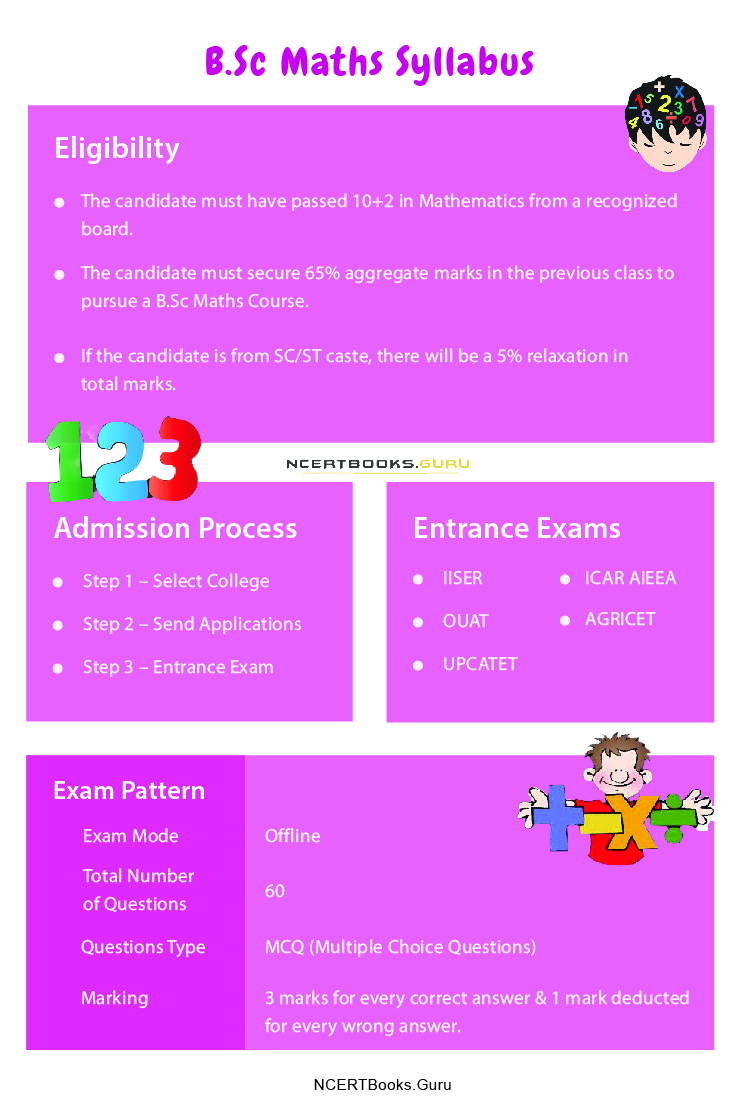# B.Sc Maths Syllabus PDF Downoad | Yearwise B.Sc Mathematics Course Structure & Exam Pattern

B.Sc Maths Syllabus: If you love solving Mathematics equations and playing with numbers, then B.Sc Maths Course is the best stream you should pick for your career. The B.Sc Maths Course stands for Bachelor of Science in Mathematics. Almost all universities and colleges across India provide B.Sc Maths, a 3 years course to those who have studied Maths in 10+2.

In B.Sc Maths, the students need to deal with structure, space, and change. If you want to pursue this course, then you have to meet the eligibility criteria as per any university or college. After pursuing this course, the student can set up their promising career ahead in various fields.

## B.Sc Mathematics Eligibility Criteria

The desirable candidate needs to follow the eligibility criteria before applying for the Course. Well, the eligibility criteria may differ from universities to colleges. Have a look at the expected standard that follows every college in India.

• The candidate must have passed 10+2 in Mathematics from a recognized board.
• The candidate must secure 65% aggregate marks in the previous class to pursue a B.Sc Maths Course.
• If the candidate is from SC/ST caste, there will be a 5% relaxation in total marks.

### B.Sc Maths Course Duration

The Course is designed in such a way that the candidate can learn and become a master in skills like algebra, calculus, and data analysis. The whole course provides in-depth knowledge of Math for three years, i.e., six semesters. The student also learns about data science and statistics.

Get to Know More about other types of Course Details in Streamwise, and Category wise.

### B.Sc Maths Course Syllabus

In the below table, we have explained the B.Sc Maths syllabus that you’ll get in the Course. With this degree course and such a high level of education, you can go for higher studies.

 Topics (Year one) Sub-Topics Calculus Differential Calculus Asymptotes Integral Calculus Multiple Integrals Geometrical application of differential equations Probability & Statistics Probability & Theoretical distributions Testing of Hypothesis Correlation & regression Statistical Quality control Transformation of Random Variable Analytical Solid Geometry Analytical geometry of two dimensions Analytical geometry of three dimensions Paraboloids Central Coincides Transformation of Rectangular axis Differential Equations Solving differential equations Formation and solving PDE Homogeneous linear differential equations Laplace transforms Differential equations with constant coefficients

 Topics (Year Two) Sub-Topics Real Analysis Real valued functions Sets & functions Real numbers Least upper bounds Equivalence and countability Abstract Algebra Group & commutative group Definition of subgroup & examples Definition of a cyclic group Permutation Preliminaries Mechanics Friction Common catenary Impact Virtual work Moment of force Linear Algebra Inner product spaces Linear transformations Eigenvalues and Eigenvectors Vector spaces Topics (Year Three) Sub-topics Discrete Mathematics Relations Division algorithms Logic Graph Theory Linear Programming & its Applications Year programming problem Problem formulation Types of solutions Linear programming in the matrix Complex Analysis Analytical functions Transformation Contour integral Taylor and Laurent Evaluation of integral Numerical Analysis Direct & iterative Method Numerical differentiation & integration Polynomial approximation Numerical solution of ordinary differential equations Numerical solution of partial differential methods

• Step 1 – Select College: The first step a candidate must do is search for the best colleges/universities offering a B.Sc Maths course. Once the list is prepared, make sure to check their Fees and Eligibility Criteria.
• Step 2 – Send Applications: If the Candidate has identified the college for him/her, the next step is to apply for the course. A candidate needs to carefully fill up all the details, and he/she should know all the details in advance.
• Step 3 – Entrance Exam: Most colleges/universities conduct entrance exams for students who want to take admission in their colleges. His/her score level will judge the student in the entrance exam along with the student’s 10+2 scores. If the Candidate has managed good marks in both, he/she will be offered admission.

• IISER
• OUAT
• UPCATET
• ICAR AIEEA
• AGRICET

Also, Check:

### How to Prepare for the B.Sc Maths Entrance Exams?

To Crack B.Sc entrance exams, the Candidate should be right in aptitude such as:

• Sets
• Linear inequalities
• Binomial Theorem
• Conic Sections
• Limits & Derivatives
• Integers
• Vectors
• Matrices
• Geometry
• Conic sections

Besides these subjects, a candidate needs to prepare for Physics and Biology subjects as well.### B.Sc Entrance Exam Pattern

 Exam Mode Online Total Number of Questions 60 Questions Type MCQ (Multiple Choice Questions) Marking 3 marks for every correct answer & 1 mark deducted for every wrong answer. Duration 3 Hours

### FAQ’s on B.Sc Maths Course

Question 1.
Which books should I refer to for the B.Sc Maths Entrance exam?

For good scores, you need the best study material. So, here is the list of books that you could use for your help.

• Problems Plus in IIT Mathematics by A. Das Gupta
• D. Lee Concise Inorganic Chemistry by O.P. Jee
• Concepts of Physics Volume by H.C Verma
• Follow NCERT books of XI and XII.

Question 2.
Does B.Sc Math is more difficult than B.A Math?

There is no significant difference between BA and B.Sc Mathematics. Both courses can help learners gain in-depth knowledge about statistics, data, regression, linear equations, and many more.

Question 3.
What jobs can I do after B.Sc Maths?